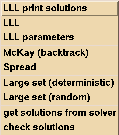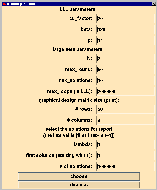´

## Solvers for diophantine equationsBy choosing "Solve" in the main window of DISCRETA, you get the menu above. Here you have the choice between different solvers for integer matrix equations.

All but one solver are implemented by Alfred Wassermann

• LLL print solutions
This method is based on lattice basis reduction (see A. K. Lenstra, H. W. Lenstra Jr. and L. Lovász) together with an exhaustive enumeration of all solutions. This solver is recommended for problems with large lambda.

• LLL
This is the same algorithm as above, with the exception that the solutions are only counted, not printed.

• LLL parameters
Here, some parameters for the lattice basis reduction and the large set algorithms (see below) can be adjusted.
• c0_factor: If this number is too small, the LLL-algorithm fails to compute a complete basis of the kernel. If this number is too large there may be an integer overflow on 32 bit computers.
• beta, p: These numbers determine the quality of the lattice basis reduction. Large values give a better lattice, at the cost of computing time.

• McKay (backtrack)
This solver was implemented by Brendan McKay and adapted to DISCRETA by Alfred Wassermann. It is very good, if the Kramer-Mesner matrix is not too big and lambda is small. Meanwhile, this method is beaten by the algorithm spread.

This solver is based on backtracking along the rows of the linear system and was proposed by Rudolf Mathon. It is the method of choice for problems with lambda=1.• Large set (deterministic)
This method computes all solutions for the required value of lambda (with the algorithm LLL). Then we apply the algorithm spread to collect a set of solutions which gives a large set. This method was proposed by Spyros Magliveras. It is feasible, if there are at most about 10000 solutions of the original system.

• Large set (random)
This method is due to an idea of Reinhard Laue: The method tries to find max_solutions solutions for the required value of lambda (with the algorithm LLL). Then one solutions out of these solutions is chosen randomly. The corresponding columns of the linear system are deleted, then we try to find a design for the required value of lambda in the remaining system. This is iterated until all columns are deleted or there are no solutions. In the first case we have found a large set, in the second case we start again from the beginning. We do this at the most max_round times. In each step we stop after max_loops loops of the LLL-algorithm.

Attention: The value N of the large set LS[N](t,k,v) and the parameters max_solutions, max_round, max_loops have to be adjusted in the submenu LLL parameter.

• The last thing to do is to check the computed solutions: first press "get solutions from solver" to get the solutions explicitely, then choose "check solutions" to check the correctness of the results.

Remark: You have to click on the pictures to get them largely.

Back to the description .
Back to the DISCRETA homepage .
Last updated: September 8, 1999, Alfred Wassermann

University of Bayreuth -